# Numbers up to 10,000,000

## NUMBERS AND OPERATIONS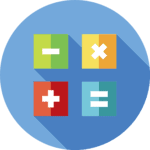A natural number (ℕ) is considered any of the numbers used to count the elements of a set or sort.

Our System of Numeration (or Numeral System) is both decimal and positional:

- Decimal, also called base ten numerals, because ten units of a lower order form a unit of the immediately higher order (10 ones equals 1 ten, 10 tens equals 1 hundred...).

- Positional, because the value of a figure will depend on the place it occupies within the number (ones, tens, hundreds...).

Furthermore, the set of natural numbers is represented by ℕ and is distributed as follows: ℕ = { 1, 2, 3, 4, 5, 6, 7, 8 , 9, ... } and, therefore, it is considered that the natural numbers, as a whole, are ordered, positive and tend to infinity.

THE NATURAL NUMBERS REGARDING THE NUMERICAL SET

We provide you with a visual scheme so that you can correctly locate the natural numbers (ℕ) within the set (also called "mathematical notation") of the integers (ℤ), rational (ℚ), real (ℝ) and complex (ℂ):Remember, mathematical symbols convey a formal language based on universal rules and represent in themselves a concept, a relationship, an operation or a mathematical formula and, therefore, should never be understood as a simple abbreviation.

#### Read and write the natural numbers up to 10,000,000

Throughout the 3rd year of Primary Education you learned to read and write four and five digit numbers (up to 99,999) and now you will discover the six and seven-digit numbers.

You will know the "millions" and the "hundred thousands" as well as the rule of the use of the comma to group the digits of three in three and as a separator from the thousands and the millions.

HOW TO READ A NATURAL SIX-DIGIT NUMBER?

Any six-digit natural number will be made up of hundreds of thousands (H th), tens of thousands (T th), ones of thousands (Th), hundreds (H), tens (T) and ones (O). We will always write a comma between the one thousands and hundreds numbers.

SPANISHENGLISHTo correctly read any whole number up to 999,999 we will first read the figures to the left of the comma (hundred thousands, ten thousands and one thousands); then we will name the comma as "thousand" and, finally, we will read the figures located to the right of the point (hundreds, tens and ones).AND HOW TO READ A NATURAL SEVEN-DIGIT NUMBER?

The seven-digit natural numbers are made up of millions (M), hundred thousands (Hth), ten thousands (Tth), thousands (Th), hundreds (H), tens (T) and ones (O).

SPANISHENGLISHIn the whole numbers of seven digits, we will write a separator comma in the following cases:

- Between the digit of millions (M) and hundred thousands (H th) to identify "millions".

- Between the digit of thousands (Th) and hundreds (H) to identify the "thousands".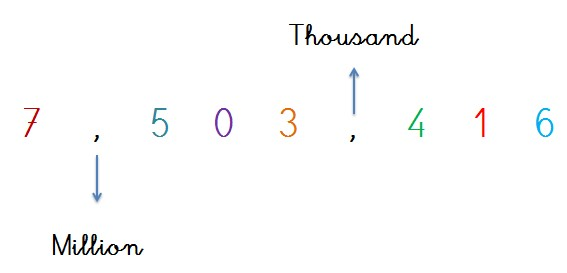To correctly read any whole number up to 9,999,999 first the digit on the left is read, then the comma is read as "million" (singular) or "millions" (plural); then the three digits located between the two commas are named as if they were a three-digit number with ones, tens and hundreds. Next, the comma is read as "thousand" and finally the three digits to the right of the comma are read.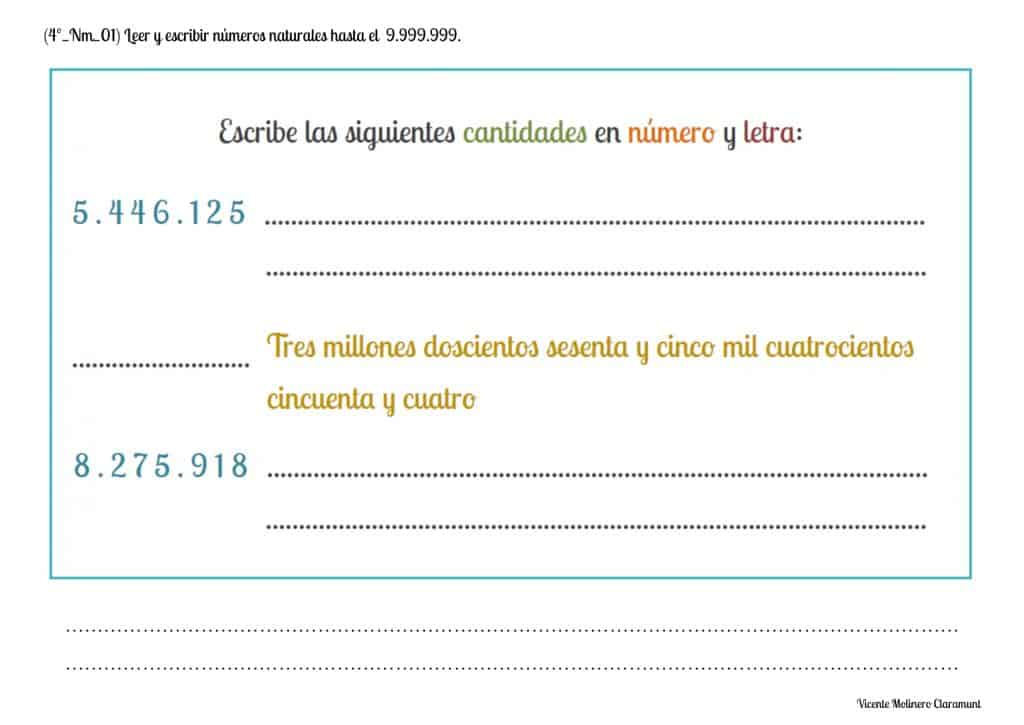#### Know the place value of figures with numbers up to 10,000,000

The place value (or position) of a number is the value that any of its digits takes according to the position it occupies within the number itself (ones, tens, hundreds...).

Very important! The change of position of a digit within a number will modify the total value of the same.

PLACE VALUE OF THE 6 AND 7 DIGIT NUMBERS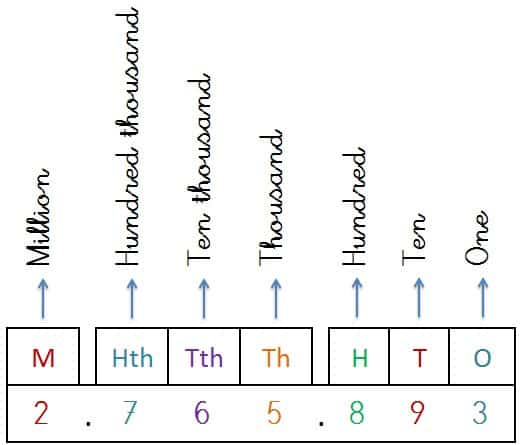- The "one" is the smallest integer element that we can use to count and represents the last digit of any whole number.

- The "ten" represents groupings of ones of 10 in 10 and corresponds to the penultimate digit of any whole number.

- The "hundred" groups the tens of 10 into 10 as well as the ones of 100 into 100; and occupies the third to last digit of any whole number.

- The "thousand" brings together the hundreds of 10 in 10, the tens of 100 in 100 and the ones of 1,000 in 1,000 being located in the fourth digit of the whole numbers.

- The "ten thousand" group the thousands in groups of 10, the hundreds in groups of 100, the tens in groups of 1,000 and the ones in groups of 10,000 being the equivalent of the fifth digit according to its place value.

- The "hundred thousand" encompasses the ten thousands of 10 in 10 , the one thousand in groups of 100, hundreds in groups of 1,000, tens in groups of 10,000 and, ones in groups of 100,000 being located in the sixth digit of the whole numbers.

- The "million" comprises the ones of 1,000,000 in 1,000,000, the tens of 100,000 in 100,000, the hundreds of 10,000 in 10,000, the thousands of 1,000 in 1,000, the ten thousands of 100 in 100 and the hundred thousands of 10 in 10. Represents the seventh digit of any natural number.

EQUIVALENCES BETWEEN 7-DIGIT NUMBERS

We present a comparative table so that you can visually understand the equivalences existing between the digits of the natural numbers of up to 7 digits: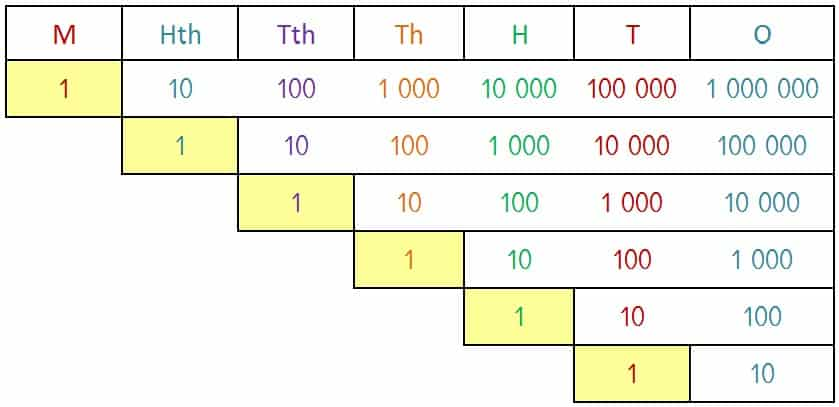#### Compose and decompose up to 10,000,000 according to the value and order of position of their digits

We offer you various printable activities so that you can quickly learn to compose and decompose the natural numbers from 0 to 10,000,000 into millions, hundred thousands, ten thousands, thousands, hundreds, tens and ones.

SPANISHENGLISH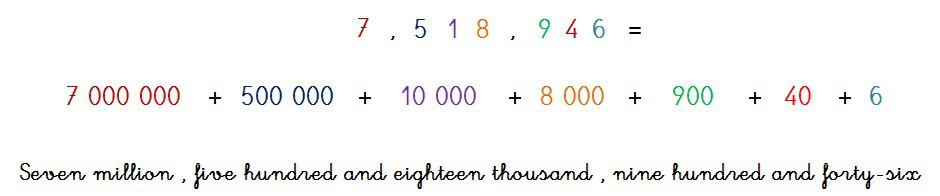#### Classify natural numbers up to 10,000,000: Greater than - Less than - Equal

We explain the concepts "greater than", "less than" and "equal to" as well as their symbols (>, <, =) with the numbers from 0 to 10,000,000. All this from a combination of printable cards accompanied by an educational game.

SPANISHENGLISH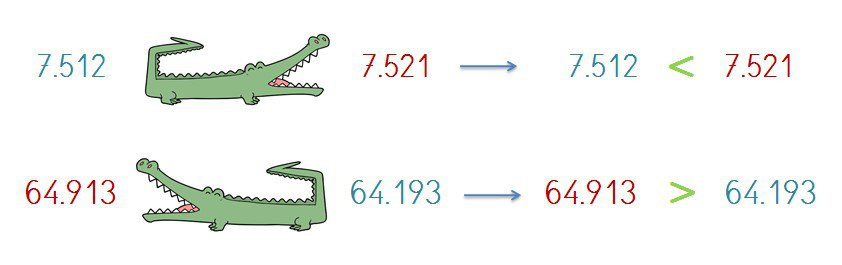#### Identify the largest and smallest numbers in a series of natural numbers up to 10,000,000

We explain how to identify which is the highest number (or biggest number) and the lowest (or smallest number) with the numbers from 1 to 10,000,000 starting from the downloadable exercises that we have prepared.

SPANISHENGLISH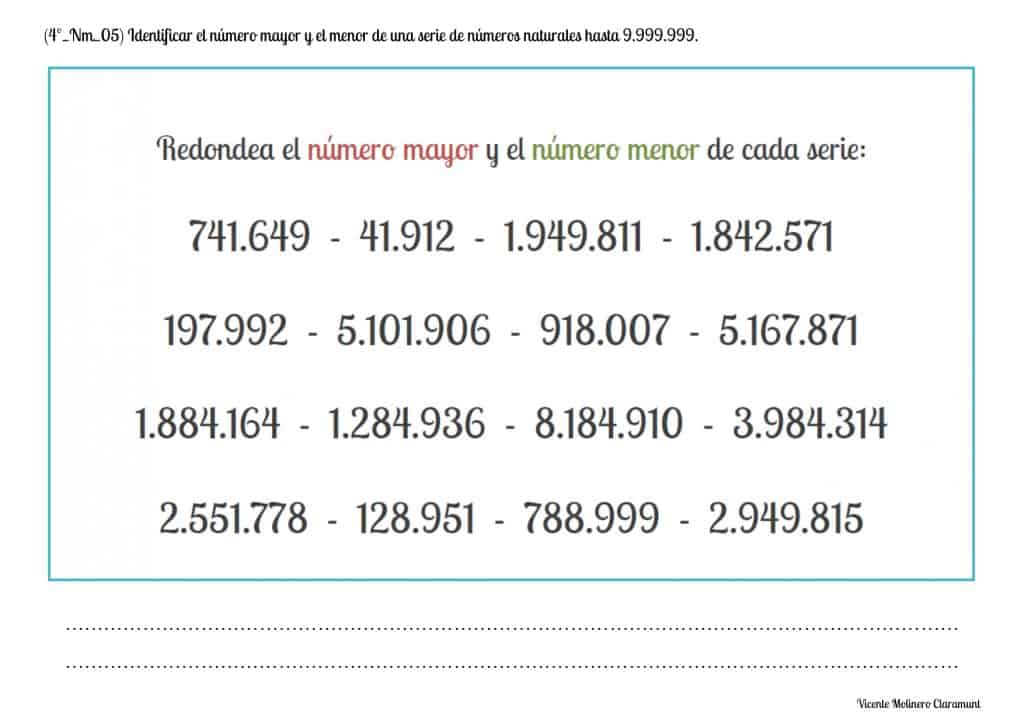#### Classify natural numbers up to 10,000,000: Before and After numbers

We provide you some PDF exercises where you have to join the natural numbers from 1 to 9,999,999 of the central column with their previous number (left) and their following number (right):

- The before number (or previous number) is the one that has one less unit than the number from which we started and, therefore, we will jump to the left in the number line.

- The after number (or next number) will be the one that has one more unit than the number we started from and, therefore, we will jump to the right in the number line.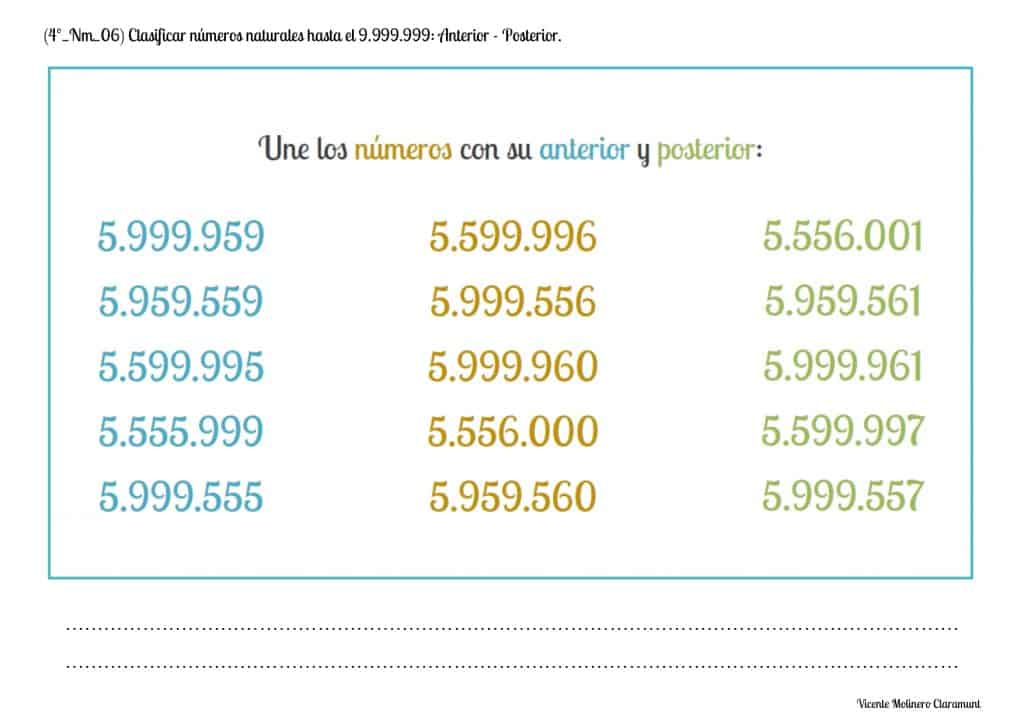#### Write the numbers before and after a given with natural numbers up to 10,000,000

We instruct you in some activities where we randomly present you natural numbers from 0 to 10,000,000 on which you must recognize and interpret their position on a number line imaginary to later write its previous number (before number) and its later number (after or next number).#### Count up natural numbers up to 10,000,000

We have created worksheets to download and print where, from a random natural number from 0 to 10,000,000 you must count up until you complete an imaginary number line.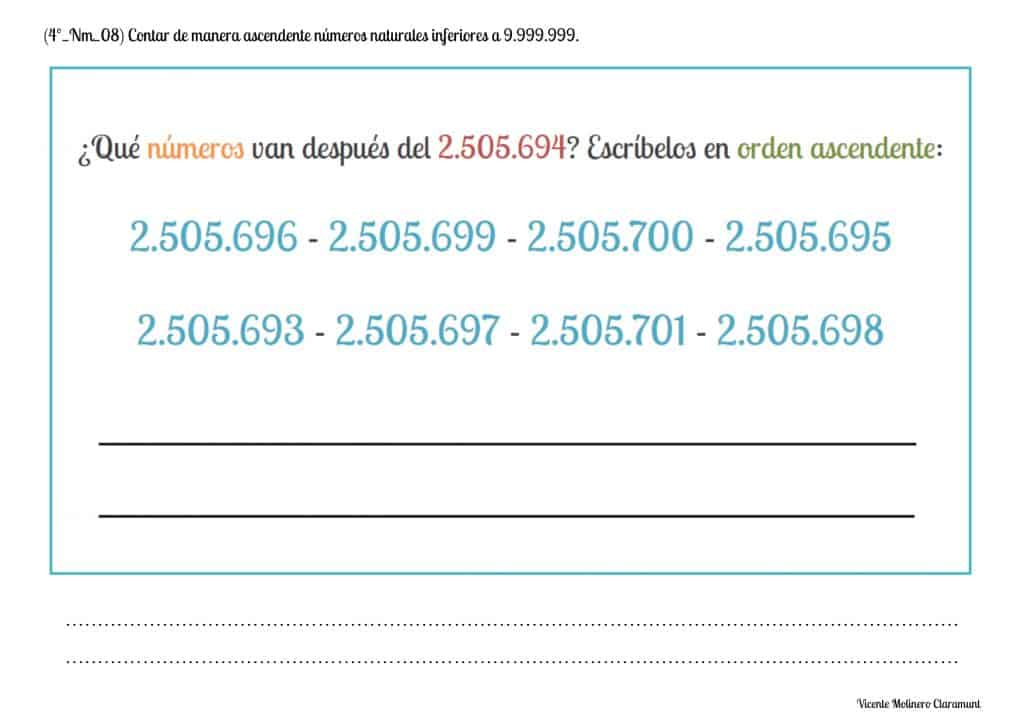#### Continue ascending series two by two, three by three... up to 10,000,000

We present some practical exercises where we provide you with numerical series using the numbers up to 10,000,000 in which, to solve them satisfactorily, you must count in ascending order giving numeric jumps two by two (2's), three by three (3's), four by four (4's)...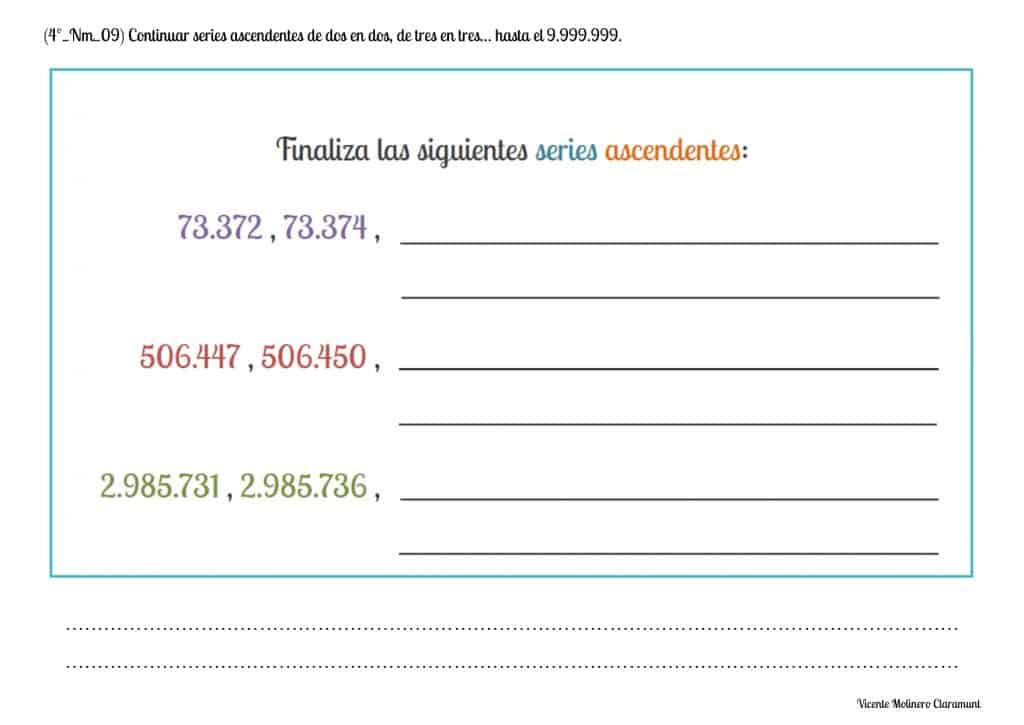#### Count down natural numbers up to 10,000,000

We have prepared some activities ready to download and print where, from a natural number from 0 to 10,000,000 you must count term by term and from form descending until completing the number line.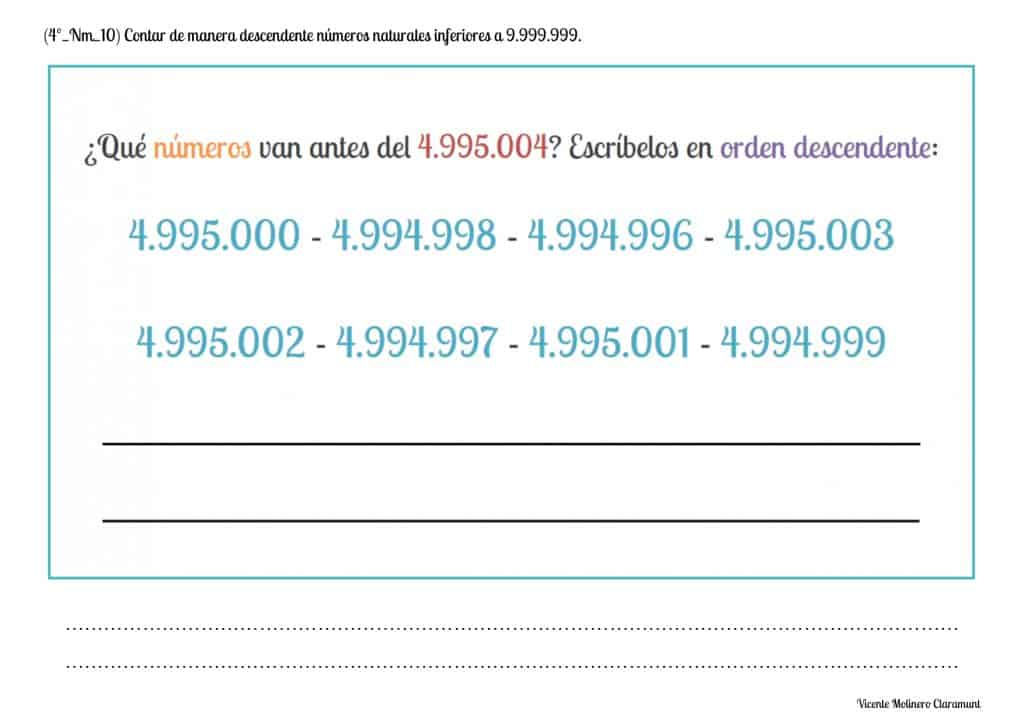#### Continue descending series two by two, three by three... up to 10,000,000

We present some funny exercises where we provide you with numerical series using the numbers up to 10,000,000 in which, to solve them correctly, you must count in descending order giving numeric jumps two by two (2's), three by three (3's), four by four (4's)...#### Order numbers up to 10,000,000 by representing on a number line

We provide you with natural numbers up to 10,000,000 unordered so that, based on their graphic representation on a number line, you can compare them and indicate which one is its exact position or order.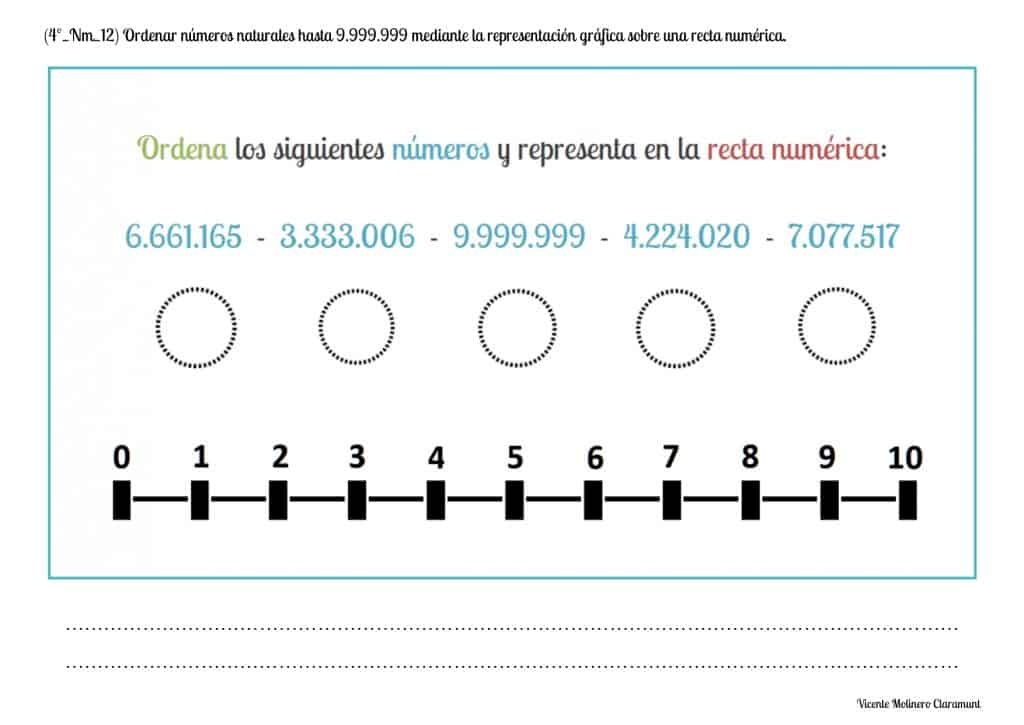#### Recognize and represent natural numbers up to 10,000,000 on abacus

Do you know what an abacus is? And could you explain what it is for? We explain it to you:

An abacus is an ancient calculation tool used to solve arithmetic operations such as addition and subtraction. In addition, today it is very useful to promote learning of numerical decomposition and the place value of any number.

Do you dare to solve the activities that we propose using the abacus?#### Approximate numbers to the one, ten and / or hundred thousand and / or the nearest million

ROUNDING TO THE NEAREST THOUSAND

For rounding off to the nearest 1,000 we will always look at the digit in the hundreds place:

- If the digit of the hundreds is 0, 1, 2, 3 or 4 (less than 5), we will approximate the thousand given, that is, thousand will remain as it appears.

- If the hundred digit is 5, 6, 7, 8 or 9 (greater than or equal to 5), we will approximate the immediately higher thousand.

SPANISHENGLISH3 , 1 8 9 is close to 3,000 because 1 < 5

4 , 9 2 5 is close to 5,000 because 9 > 5

ROUNDING TO THE NEAREST TEN THOUSAND

For rounding off to the nearest 10,000 we will always look at the digit in the thousands place:

- If the digit of the thousands is 0, 1, 2, 3 or 4 (less than 5), we will approximate the ten thousand given, that is, ten thousand will remain as it appears.

- If the thousand digit is 5, 6, 7, 8 or 9 (greater than or equal to 5), we will approximate the immediately higher ten thousand.

SPANISHENGLISH6 2 , 9 5 7 is close to 60,000 because 2 < 5

1 8 , 3 0 2 is close to 20,000 because 8 > 5

ROUNDING TO THE NEAREST HUNDRED THOUSAND

For rounding off to the nearest 100,000 we will always look at the digit in the ten thousands place:

- If the digit of the ten thousands is 0, 1, 2, 3 or 4 (less than 5), we will approximate the hundred thousand given, that is, hundred thousand will remain as it appears.

- If the ten thousand digit is 5, 6, 7, 8 or 9 (greater than or equal to 5), we will approximate the immediately higher hundred thousand.

SPANISHENGLISH6 3 5 , 0 8 9 is close to 600,000 because 3 < 5

8 5 2 , 9 9 5 is close to 900,000 because 5 = 5

ROUNDING TO THE NEAREST MILLION

For rounding off to the nearest 1,000,000 we will always look at the digit in the hundred thousands place:

- If the digit of the hundred thousands is 0, 1, 2, 3 or 4 (less than 5), we will approximate the million given, that is, million will remain as it appears.

2 , 4 0 8 , 5 7 6 is close to 2,000,000 because 4 < 5

- If the hundred thousand digit is 5, 6, 7, 8 or 9 (greater than or equal to 5), we will approximate the immediately higher million.

7 , 6 9 5 , 3 1 4 is close to 8,000,000 because 6 > 5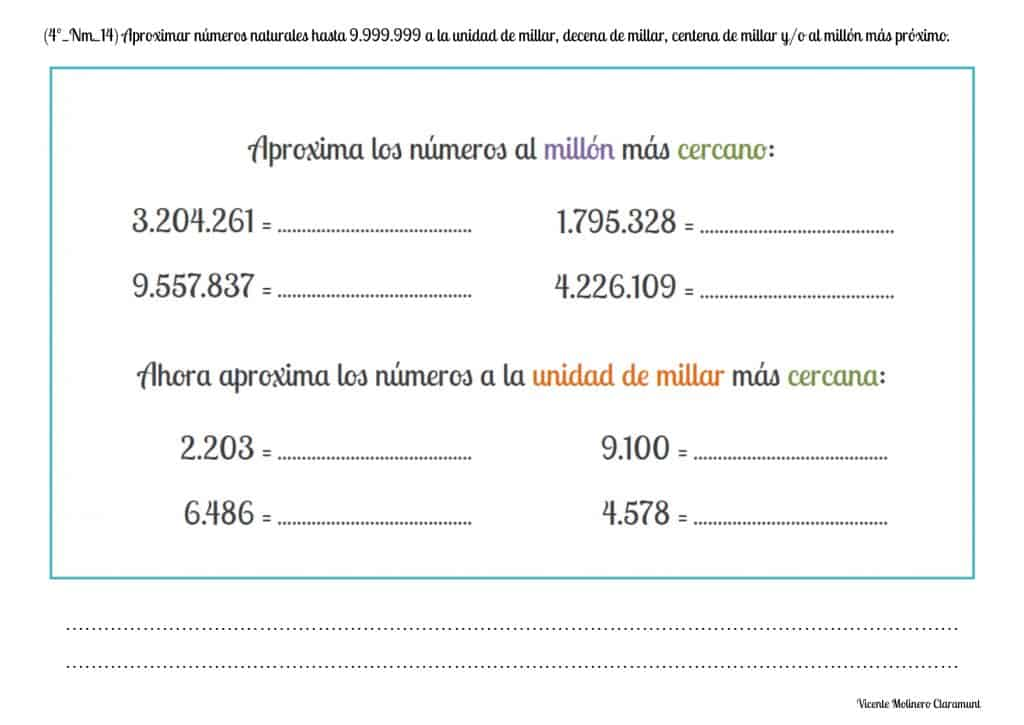#### Recognize odd and even natural numbers up to 10,000,000

Do you know what even numbers and odd numbers are? And could you explain how the even numbers differ from the odd numbers?

The even natural numbers are those that end in 0, 2, 4, 6 and 8 while the odd natural numbers are those that end in 1, 3, 5, 7 and 9.

Concentrate on your work and try hard and you will see how you will become a great mathematician.Select more exercises, worksheets and activities of Mathematics for each of the 4 learning blocks of the Educational Curriculum ("Numbers and Operations", "Quantities and Measurements", "Geometric and Spatial Reasoning" and "Data Analysis and Probability") aimed at improving the logical-mathematical competencies and skills that are developed throughout "Grade 4" or "Year 5":